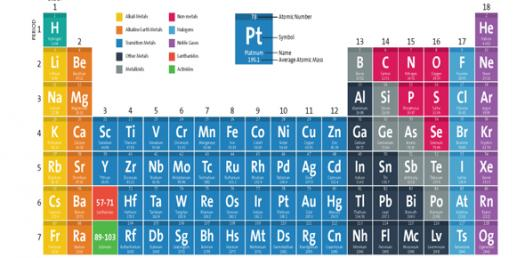# Periodic Table Vocabulary Quiz!

10 Questions | Attempts: 427
ShareSettings• 1.
What are the terms for rows and columns on the periodic table?
• A.

Groups and periods

• B.

Rows and columns

• C.

Rows and groups

• D.

Periods and columns

• E.

Rows and periods

• F.

Columns and groups

• 2.
What does the symbol in the upper left-hand corner stand for?
• A.

Atomic Number

• B.

Mass of the Element

• C.

How many electrons

• 3.
Which is equivilant to the atomic number?
• A.

Protons

• B.

Orbits

• C.

Electrons

• 4.
What are the large letters on the elements?
• A.

Large letters

• B.

Chemical symbol

• C.

Atomic number

• 5.
Where is the most of the mass located in an atom?
• A.

The protons

• B.

The protons and electrons

• C.

The electrons

• D.

The electrons and neutrons

• E.

The neutrons and protons

• 6.
Each atom has a nucleus which contains _______ which are positively charged.
• 7.
A(n) ____ is the smallest part of an element that has all of the properties of that element.
• 8.
Orbiting the nucleus of an atom are negatively charged particles called ____.
• 9.
Each atom has a nucleus which contains _______ which are not charged.
• 10.
The number of protons plus the number of neutrons determines an atoms ___.

## Related TopicsBack to top
×

Wait!
Here's an interesting quiz for you.